# 深入浅出 Tensorflow（三）：训练神经网络模型的常用方法

2017 年 5 月 03 日

## 梯度下降算法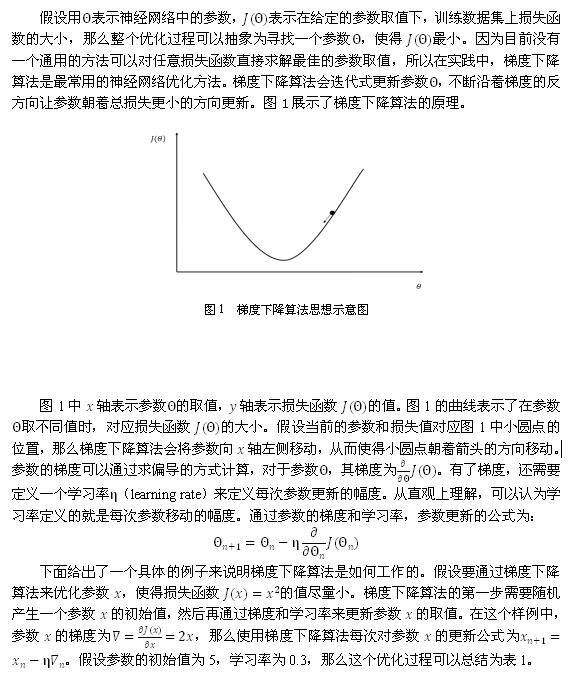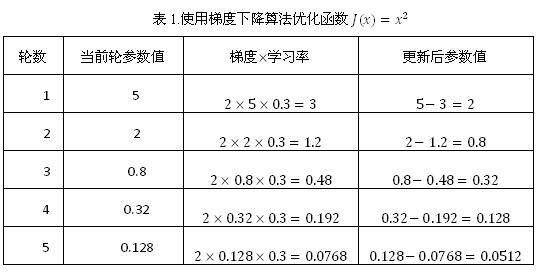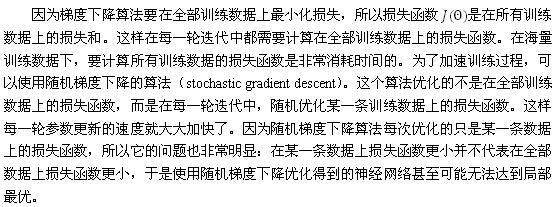### 学习率的设置

1

5

2*5*1=10

5-10=-5

2

-5

2*(-5)*1=-10

-5-(-10)=5

3

5

2*5*1=10

5-10=-5

 decayed_learning_rate =    learning_rate * decay_rate ^ (global_step / decay_ steps)

  # 通过 exponential_decay 函数生成学习率。learning_rate = tf.train.exponential_decay(learning_rate_base, global_step, decay_step, decay_rate)  # 使用指数衰减的学习率。在 minimize 函数中传入 global_step 将自动更新 # global_step 参数，从而使得学习率也得到相应更新。learning_step =    tf.train.GradientDescentOptimizer(learning_rate)\     .minimize(...my loss..., global_step=global_step)  

### 过拟合问题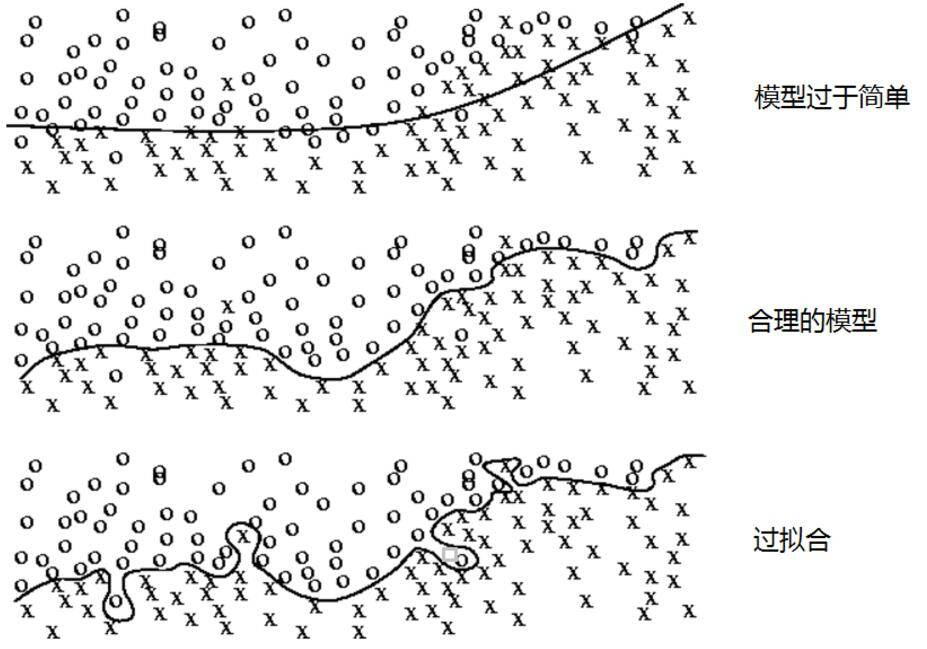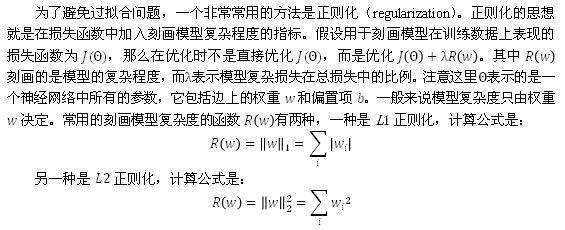w= tf.Variable(tf.random_normal([2, 1], stddev=1, seed=1))y = tf.matmul(x, w) loss = tf.reduce_mean(tf.square(y_ - y)) +        tf.contrib.layers.l2_regularizer(lambda)(w)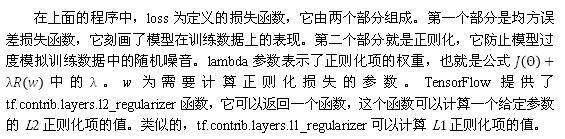### 滑动平均模型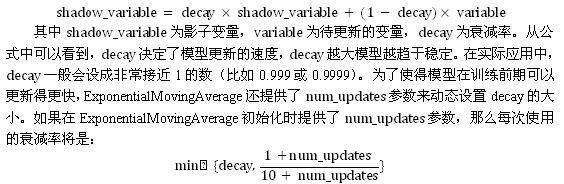import tensorflow as tf v1 = tf.Variable(0.0, dtype=tf.float32)step = tf.Variable(0, trainable=False) # 定义一个滑动平均的类（class）。初始化时给定了衰减率（0.99）和控制衰减率的变量 step。ema = tf.train.ExponentialMovingAverage(0.99, step)# 定义一个更新变量滑动平均的操作。这里需要给定一个列表，每次执行这个操作时# 这个列表中的变量都会被更新。maintain_averages_op = ema.apply([v1])                            with tf.Session() as sess:   init_op = tf.initialize_all_variables()sess.run(init_op)    # 更新变量 v1 的值到 5。sess.run(tf.assign(v1, 5))   # 更新 v1 的滑动平均值。衰减率为 min{0.99,(1+step)/(10+step)= 0.1}=0.1,   # 所以 v1 的滑动平均会被更新为 0.10+0.95=4.5。sess.run(maintain_averages_op)   print sess.run([v1, ema.average(v1)])        #  输出 [5.0, 4.5]       # 更新 step 的值为 10000。   sess.run(tf.assign(step, 10000))     # 更新 v1 的值为 10。sess.run(tf.assign(v1, 10))   # 更新 v1 的滑动平均值。衰减率为 min{0.99,(1+step)/(10+step)  0.999}=0.99,   # 所以 v1 的滑动平均会被更新为 0.994.5+0.0110=4.555。   sess.run(maintain_averages_op)print sess.run([v1, ema.average(v1)])      #  输出 [10.0, 4.5549998]

#### ensorFlow 最佳实践样例程序

# -*- coding: utf-8 -*-import tensorflow as tf # 定义神经网络结构相关的参数。INPUT_NODE = 784  OUTPUT_NODE = 10  LAYER1_NODE = 500  # 通过 tf.get_variable 函数来获取变量。在训练神经网络时会创建这些变量；在测试时会通# 过保存的模型加载这些变量的取值。而且更加方便的是，因为可以在变量加载时将滑动平均变量# 重命名，所以可以直接通过同样的名字在训练时使用变量自身，而在测试时使用变量的滑动平# 均值。在这个函数中，将变量的正则化损失加入损失集合。def get_weight_variable(shape, regularizer):  weights = tf.get_variable(      "weights", shape,        initializer=tf.truncated_normal_initializer(stddev=0.1))   # 当给出了正则化生成函数时，将当前变量的正则化损失加入名字为 losses 的集合。在这里  # 使用了 add_to_collection 函数将一个张量加入一个集合，而这个集合的名称为 losses。  # 这是自定义的集合，不在 TensorFlow 自动管理的集合列表中。   if regularizer != None:        tf.add_to_collection('losses', regularizer(weights))   return weights # 定义神经网络的前向传播过程。def inference(input_tensor, regularizer):   # 声明第一层神经网络的变量并完成前向传播过程。   with tf.variable_scope('layer1'):      # 这里通过 tf.get_variable 或 tf.Variable 没有本质区别，因为在训练或是测试中      # 没有在同一个程序中多次调用这个函数。如果在同一个程序中多次调用，在第一次调用      # 之后需要将 reuse 参数设置为 True。      weights = get_weight_variable(          [INPUT_NODE, LAYER1_NODE], regularizer)      biases = tf.get_variable(          "biases", [LAYER1_NODE],           initializer=tf. constant_initializer(0.0))      layer1 = tf.nn.relu(tf.matmul(input_tensor, weights) + biases)    # 类似的声明第二层神经网络的变量并完成前向传播过程。   with tf.variable_scope('layer2'):       weights = get_weight_variable(            [LAYER1_NODE, OUTPUT_NODE], regularizer)        biases = tf.get_variable(           "biases", [OUTPUT_NODE],            initializer=tf. constant_initializer(0.0))       layer2 = tf.matmul(layer1, weights) + biases     # 返回最后前向传播的结果。   return layer2

# -*- coding: utf-8 -*-import os import tensorflow as tffrom tensorflow.examples.tutorials.mnist import input_data # 加载 mnist_inference.py 中定义的常量和前向传播的函数。import mnist_inference # 配置神经网络的参数。BATCH_SIZE = 100 LEARNING_RATE_BASE = 0.8LEARNING_RATE_DECAY = 0.99REGULARAZTION_RATE = 0.0001TRAINING_STEPS = 30000MOVING_AVERAGE_DECAY = 0.99 # 模型保存的路径和文件名。MODEL_SAVE_PATH = "/path/to/model/"MODEL_NAME = "model.ckpt" def train(mnist):  # 定义输入输出 placeholder。   x = tf.placeholder(       tf.float32, [None, mnist_inference.INPUT_NODE], name='x-input')   y_ = tf.placeholder(       tf.float32, [None, mnist_inference.OUTPUT_NODE], name='y-input')      regularizer = tf.contrib.layers.l2_regularizer(REGULARAZTION_RATE)   # 直接使用 mnist_inference.py 中定义的前向传播过程。   y = mnist_inference.inference(x, regularizer)   global_step = tf.Variable(0, trainable=False)   # 定义损失函数、学习率、滑动平均操作以及训练过程。   variable_averages = tf.train.ExponentialMovingAverage(       MOVING_AVERAGE_DECAY, global_step)   variables_averages_op = variable_averages.apply(       tf.trainable_variables())   cross_entropy = tf.nn.sparse_softmax_cross_entropy_with_logits(       logits=y, labels=tf.argmax(y_, 1))   cross_entropy_mean = tf.reduce_mean(cross_entropy)   loss = cross_entropy_mean + tf.add_n(tf.get_collection('losses'))   learning_rate = tf.train.exponential_decay(       LEARNING_RATE_BASE,        global_step,        mnist.train.num_examples / BATCH_SIZE, LEARNING_RATE_DECAY)   train_step = tf.train.GradientDescentOptimizer(learning_rate)\                     .minimize(loss, global_step=global_step)     with tf.control_dependencies([train_step, variables_averages_op]):       train_op = tf.no_op(name='train')    # 初始化 TensorFlow 持久化类。   saver = tf.train.Saver()   with tf.Session() as sess:       tf.global_variables_initializer().run()        # 在训练过程中不再测试模型在验证数据上的表现，验证和测试的过程将会有一个独       # 立的程序来完成。       for i in range(TRAINING_STEPS):           xs, ys = mnist.train.next_batch(BATCH_SIZE)           _, loss_value, step = sess.run([train_op, loss, global_step],                                                   feed_dict={x: xs, y_: ys})           # 每 1000 轮保存一次模型。           if i % 1000 == 0:                 # 输出当前的训练情况。这里只输出了模型在当前训练 batch 上的损失函                 # 数大小。通过损失函数的大小可以大概了解训练的情况。在验证数据集上的                 # 正确率信息会有一个单独的程序来生成。               print("After %d training step(s), loss on training "                        "batch is %g." % (step, loss_value))                 # 保存当前的模型。注意这里给出了 global_step 参数，这样可以让每个被                 # 保存模型的文件名末尾加上训练的轮数，比如“model.ckpt-1000”表示                      # 训练 1000 轮之后得到的模型。               saver.save(                      sess, os.path.join(MODEL_SAVE_PATH, MODEL_NAME),                      global_step=global_step) def main(argv=None):    mnist = input_data.read_data_sets("/tmp/data", one_hot=True)   train(mnist) if __name__ == '__main__':   tf.app.run()

 ~/mnist$python mnist_train.py Extracting /tmp/data/train-images-idx3-ubyte.gzExtracting /tmp/data/train-labels-idx1-ubyte.gzExtracting /tmp/data/t10k-images-idx3-ubyte.gzExtracting /tmp/data/t10k-labels-idx1-ubyte.gzAfter 1 training step(s), loss on training batch is 3.46893.After 1001 training step(s), loss on training batch is 0.172291.After 2001 training step(s), loss on training batch is 0.197483.After 3001 training step(s), loss on training batch is 0.153582.After 4001 training step(s), loss on training batch is 0.117219.After 5001 training step(s), loss on training batch is 0.121872.After 6001 training step(s), loss on training batch is 0.0976607. 在新的训练代码中，不再将训练和测试跑在一起。训练过程中，每 1000 轮输出一次在当前训练 batch 上损失函数的大小来大致估计训练的效果。在上面的程序中，每 1000 轮保存一次训练好的模型，这样可以通过一个单独的测试程序，更加方便地在滑动平均模型上做测试。以下代码给出了测试程序 mnist_eval.py。 复制代码 # -*- coding: utf-8 -*-import timeimport tensorflow as tffrom tensorflow.examples.tutorials.mnist import input_data # 加载 mnist_inference.py 和 mnist_train.py 中定义的常量和函数。import mnist_inferenceimport mnist_train # 每 10 秒加载一次最新的模型，并在测试数据上测试最新模型的正确率。EVAL_INTERVAL_SECS = 10 def evaluate(mnist): with tf.Graph().as_default() as g: # 定义输入输出的格式。 x = tf.placeholder( tf.float32, [None, mnist_inference.INPUT_NODE], name='x-input') y_ = tf.placeholder( tf.float32, [None, mnist_inference.OUTPUT_NODE], name='y-input') validate_feed = {x: mnist.validation.images, y_:mnist.validation. labels} # 直接通过调用封装好的函数来计算前向传播的结果。因为测试时不关注正则化损失的值， # 所以这里用于计算正则化损失的函数被设置为 None。 y = mnist_inference.inference(x, None) # 使用前向传播的结果计算正确率。如果需要对未知的样例进行分类，那么使用 # tf.argmax(y, 1) 就可以得到输入样例的预测类别了。 correct_prediction = tf.equal(tf.argmax(y, 1), tf.argmax(y_, 1)) accuracy = tf.reduce_mean(tf.cast(correct_prediction, tf.float32)) # 通过变量重命名的方式来加载模型，这样在前向传播的过程中就不需要调用求滑动平均 # 的函数来获取平均值了。这使得我们可以完全共用 mnist_inference.py 中定义的 # 前向传播过程。 variable_averages = tf.train.ExponentialMovingAverage( mnist_train.MOVING_AVERAGE_DECAY) variables_to_restore = variable_averages.variables_to_restore() saver = tf.train.Saver(variables_to_restore) # 每隔 EVAL_INTERVAL_SECS 秒调用一次计算正确率的过程以检测训练过程中正确率的# 变化。 while True: with tf.Session() as sess: # tf.train.get_checkpoint_state 函数会通过 checkpoint 文件自动 # 找到目录中最新模型的文件名。 ckpt = tf.train.get_checkpoint_state( mnist_train.MODEL_SAVE_PATH) if ckpt and ckpt.model_checkpoint_path: # 加载模型。 saver.restore(sess, ckpt.model_checkpoint_path) # 通过文件名得到模型保存时迭代的轮数。 global_step = ckpt.model_checkpoint_path .split('/')[-1].split('-')[-1] accuracy_score = sess.run(accuracy, feed_dict=validate_feed) print("After %s training step(s), validation " "accuracy = %g" % (global_step, accuracy_score)) else: print('No checkpoint file found') return time.sleep(EVAL_INTERVAL_SECS) def main(argv=None): mnist = input_data.read_data_sets("/tmp/data", one_hot=True) evaluate(mnist) if __name__ == '__main__': tf.app.run() 上面给出的 mnist_eval.py 程序会每隔 10 秒运行一次，每次运行都是读取最新保存的模型, 并在 MNIST 验证数据集上计算模型的正确率。如果需要离线预测未知数据的类别（比如这个样例程序可以判断手写体数字图片中所包含的数字），只需要将计算正确率的部分改为答案输出即可。运行 mnist_eval.py 程序可以得到类似下面的结果。注意因为这个程序每 10 秒自动运行一次，而训练程序不一定每 10 秒输出一个新模型，所以在下面的结果中会发现有些模型被测试了多次。一般在解决真实问题时，不会这么频繁地运行评测程序。 复制代码  ~/mnist$ python mnist_eval.py Extracting /tmp/data/train-images-idx3-ubyte.gzExtracting /tmp/data/train-labels-idx1-ubyte.gzExtracting /tmp/data/t10k-images-idx3-ubyte.gzExtracting /tmp/data/t10k-labels-idx1-ubyte.gzAfter 1 training step(s), validation accuracy = 0.0616After 1001 training step(s), validation accuracy = 0.9764After 2001 training step(s), validation accuracy = 0.9834After 2001 training step(s), validation accuracy = 0.9834After 3001 training step(s), validation accuracy = 0.9852After 4001 training step(s), validation accuracy = 0.9854After 5001 training step(s), validation accuracy = 0.986After 6001 training step(s), validation accuracy = 0.9854

## 作者介 **** 绍

2017 年 5 月 03 日 17:0744429

## 评论 3 条评论2019 年 04 月 08 日 22:342019 年 03 月 20 日 22:14

2019 年 03 月 20 日 22:27

• ###### 关于 TensorFlow 2.0 你需要了解的一切

起初，TensorFlow的主要目的是为构建神经网络提供高性能API。然而，借助机器学习社区对它的兴趣及时间优势，这个类库演变成一个完整的ML生态系统。

本文为下篇。

• ###### 36 丨数据分析算法篇答疑

我总结了算法篇中经常遇到的问题，精选了几个作为答疑。没有列出的问题，我也会在评论区陆续解答。

2019 年 3 月 6 日

• ###### TensorFlow 工程实战（四）：使用带注意力机制的模型分析评论者是否满意

如何利用tf.keras接口搭建一个只带有注意力机制的模型，进而实现文本分类？

• ###### 如何用向量和参数方程描述曲线？

不论我们用什么图形系统绘图，图形的呈现都离不开曲线。那我们该如何描述曲线呢？

2020 年 7 月 6 日

• ###### 深入浅出 TensorFlow（二）：TensorFlow 解决 MNIST 问题入门

本文是整个系列的第二篇文章，将会简单介绍TensorFlow安装方法、TensorFlow基本概念、神经网络基本模型，并在MNIST数据集上使用TensorFlow实现一个简单的神经网络。

• ###### Yann LeCun 都推荐的深度学习资料合集

本文是GitHub上的一个项目，包含了几乎人工智能领域全部的资料合集。

• ###### Tensorflow 图像分类从模型自定义到测试

一文详解 Tensorflow。

• ###### Embedding+MLP：如何用 TensorFlow 实现经典的深度学习模型？

你知道微软在2016年提出的深度学习模型Deep Crossing吗？它就属于经典的Embedding+MLP模型。

2020 年 11 月 18 日

• ###### 19 人工神经网络 | 各人自扫门前雪：径向基函数神经网络

在神经科学中，什么是“感受野”？如何训练径向基函数神经网络？

2018 年 1 月 20 日

• ###### 与 DeepLearning 的初次接触（上）

本文因内容较多，故分为上下两篇，此篇为上篇。

• ###### 深入浅出 Tensorflow（四）：卷积神经网络

本文将介绍卷积神经网络，通过与传统算法的对比、卷积神经网络的结构分析等方面来介绍卷积神经网络模型，并给出通过TensorFlow在MNIST数据集上实现卷积神经网络的方法。

• ###### 分布式 tensorflow 源码解读 2：MonitoredTrainingSession

MonitoredTrainingSession是tensorflow管理分布式训练中一个使用很广泛的API

• ###### 如果关注排序效果，那么这个模型可以帮到你

矩阵分解在推荐系统中的地位非常崇高，恐怕本专栏介绍的其他算法模型都不能轻易地撼动它。

2018 年 3 月 30 日

• ###### 用机器学习分析流行音乐（三）：构建模型

在本文中，我将构建不同的预测模型，并比较结果。

2019 年 3 月 4 日

• ###### 深入浅出 TensorFlow（六）TensorFlow 高层封装

为了让TensorFlow使用起来更加灵活，更加方便，可以使用一些高级封装。本文将介绍TensorFlow的四种主要封装：TensorFlow-Slim、tf.contrib.learn（之前也被称为skflow）、TFLearn、Keras；同时还将通过其中常用的三种方式在MNIST数据集上实现卷积神经网络。

Java

#### 四面美团开发岗，成功斩获offer，分享个人面经